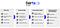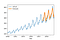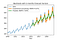# Motivation

If you are a data scientist working with time series you already know this: time series are special beasts. With regular tabular data, you can often just use scikit-learn for doing most ML things — from preprocessing to prediction and model selection. But with time series, the story is different. You can easily end up in situations where you need one library for pre-processing (e.g. Pandas to interpolate missing values and re-sample), another to detect seasonality (e.g. statsmodels), a third one to fit a forecasting model (e.g. Facebook Prophet), and finally more often than not you’ll have to implement your own backtesting and model selection routines. This can be quite tedious, as most libraries use different APIs and data types. And that’s not even mentioning cases involving more complex models based on neural networks, or problems involving external data and more dimensions. In such cases you’d likely have to implement the models yourself for your use-case, for instance using libraries such as Tensorflow or PyTorch. Overall, we feel that the experience of doing machine learning on time series in Python is just not really smooth, yet.

We are big fans of the scikit-learn approach: a single open-source library with consistent API that contains a great set of tools for end-to-end machine learning. Darts attempts to be a scikit-learn for time series, and its primary goal is to simplify the whole time series machine learning experience.Darts is an attempt to smooth the end-to-end time series machine learning experience in Python

# Show Me!

`darts` is open source and available here. You can install it in your favourite Python environment as follows:

`pip install u8darts`
`import pandas as pdfrom darts import TimeSeriesdf = pd.read_csv('AirPassengers.csv')series = TimeSeries.from_dataframe(df, 'Month', '#Passengers')`
`from darts.models import ExponentialSmoothingtrain, val = series.split_before(pd.Timestamp('19580101'))model = ExponentialSmoothing()model.fit(train)prediction = model.predict(len(val))`
`import matplotlib.pyplot as pltseries.plot(label='actual')prediction.plot(label='forecast', lw=3)plt.legend()`Forecasting the number of air passengers over 3 years (36 monthly values), using a simple exponential smoothing model.

# A Few More Details

As you may have guessed, we are mimicking the scikit-learn `fit()` and `predict()` pattern for training models and making forecasts. The `fit()`function takes in argument a training `TimeSeries` and the `predict()` function returns a new `TimeSeries` representing the forecast. This means that models manipulate `TimeSeries`, and this is pretty much the only data type being manipulated in Darts. This allows users to easily swap and compare models. For example, we could have just as easily used an auto-ARIMA model (which behind the scenes wraps around pmdarima):

`from darts.models import AutoARIMAmodel_aarima = AutoARIMA()model_aarima.fit(train)prediction_aarima = model_aarima.predict(len(val))`
• Unified `fit()` and `predict()` interface across all forecasting models, from ARIMA to neural networks.
• Models consume and produce `TimeSeries`, which means for instance that it is easy to have a regression model consume the output of a forecasting model.
• `TimeSeries` can be either univariate (1-dimensional) or multivariate (multi-dimensional). Certain models such as those based on neural nets operate on multivariate series, while others are restricted to univariate series.
• Immutability: the `TimeSeries` class is designed to be immutable.

# Another Example — Backtesting

In our example above, we used Darts to obtain once a forecast over the next 36 months starting in January 1958. However, forecasts often need to be updated as soon as new data becomes available. With Darts, it’s easy to compute the forecasts resulting from such a process, using backtesting. For instance, using backtesting to compare two models looks as follows:

`from darts.backtesting import backtest_forecastingfrom darts.models import Prophetmodels = [ExponentialSmoothing(), Prophet()]backtests = [backtest_forecasting(series,                                  model,                                  pd.Timestamp('19550101'),                                  fcast_horizon_n=3)             for model in models]`
`from darts.metrics import mapeseries.plot(label='data')for i, m in enumerate(models):    err = mape(backtests[i], series)    backtests[i].plot(lw=3, label='{}, MAPE={:.2f}%'.format(m, err))plt.title('Backtests with 3-months forecast horizon')plt.legend()`Backtesting forecasting models — here we simulate making forecasts with a 3 months horizon, every month starting in January 1955 (so the first forecast value is for April 1955).

# What Next?

We are actively developing Darts and adding new features. For instance here are a few things we would like to add:

• Time series embeddings and clustering

Written by

Written by

## Julien Herzen

#### Data scientist @ Unit8